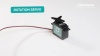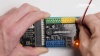## Lesson 5 – Rotation Servos

#### Hummingbird Components

1 Rotation Servo, 1 Tri-Color LED

#### Python Concepts

Generating random numbers

#### Teacher Materials

In the previous lesson, you learned to use the position servo, which moves to a particular angle. In this lesson, you will learn to use the rotation servo. The rotation servo is a motor that can rotate at different speeds.Plug in the rotation servo to SERVOS port 1 on the Bit. Make sure the black wire is aligned to ‘-,’ the red wire to ‘+,’ and the white wire to ‘S.’To set the speed of the  rotation servo, use the setRotationServo() method. This method requires two parameters. The first parameter is the port number of the servo (1-4), and the second is a speed from -100 to 100. A speed of 0 turns the motor off, while ±100 sets the motor to its maximum speed.#### Exercise 1

Try out this code. It should make the rotation servo turn at full speed for one second. Then try out other speeds. What does a negative speed do?#### Exercise 2

Write a program that makes the servo spin clockwise quickly for 2 seconds, then counterclockwise slowly for 3 seconds.

#### Exercise 3

Write a program that makes the motor run then stop 10 times.

To make the behavior of your robot less predictable, you may want to incorporate some variation so that your robot does something slightly different each time you run the program. To do this, you can use a random number generator. A random number generator is a function that picks a random number each time you call it. In Python, use randint() to pick an integer. This function takes two parameters that define the range of numbers that you are interested in. The first is the lowest number that should be generated, and the second parameter is the highest number that should be generated. For example, this line of code will generate a random number from 0 to 5.To use the randint() function, you must import it from the random library by placing this line of code at the beginning of your program.#### Exercise 4

What do you think this code will do? Make a hypothesis, and then try it out. Remember to import the random library before using the randint() function.#### Exercise 5

Modify the code from Exercise 4 to set the rotation servo to a random speed between -100 and 100.

#### Exercise 6

Connect a tri-color LED to your Hummingbird. Using the randint() function to set the tri-color LED to a random color. Then use a loop to set the LED to a random color 5 times.

#### Extra Challenge

The random library also includes other random number generators. For example, random.random() generates a random number between 0.0 and 1.0 (not including 1.0). Use this function to modify your code from Exercise 6 so that the LED stays each color for a random number of seconds between 0 and 1. Can you then make the LED stay each color for a random number of seconds between 0 and 5? Note: random.random() does not take any arguments.#### Extra Challenge

Now that you know how to use the rotation servo, try building and programming a rover! Note that the two rotation servos on the rover point in opposite directions. This means that to move the rover forward, the two servos must move in opposite directions.